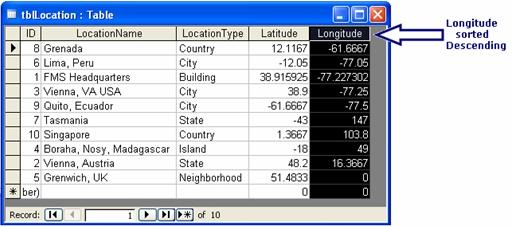# Avoid Using Decimal Field Size in Microsoft Access 2003 Tables

#### Provided by Molly Pell, Senior Systems Analyst

This problem was originally reported with Microsoft Access 2003 and remains a problem in Access 2003. This is not a problem with Access 2007 and later.

## Introduction: Number Fields Can Have Field Size Set to Decimal

In Microsoft Access tables, the data type Number has many Field Size options. Among the available Field Sizes is Decimal:Decimal fields have decimal precision of 28, and store numbers from –10^28–1 through 10^28–1 (or –10^38–1 through 10^38–1 in ADPs).

## Problems with Decimal Field Sizes in Microsoft Access 2003

Unfortunately, Decimal fields have several known issues that limit their usefulness and they should be avoided whenever possible. The main issue is with sorting decimal fields in descending order. Zeros and negative values are the sorted incorrectly, as demonstrated in the screenshot below:In the above example, negative values appear before positive values, and 0 appears at the end of the list. This issue is documented in Microsoft's KB article 837148.

In addition to the sorting problem, there are several other problems with Decimal types, including:

• Decimal types use more storage space than other numeric field types.
• Exporting tables with Decimal fields to a text file fails (Microsoft KB article 263946)
• There is no Decimal data type in VBA, so there is not a way to declare a constant with this type

## Use the Double Field Size Instead of Decimal

The best solution is to avoid using Decimal field size altogether. Instead, use the Double field size for numeric fields that require decimal values.